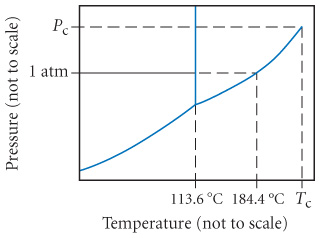# Problem: Consider the phase diagram for iodine shown below and answer each of the following questions.What is the melting point for iodine at 1 atm?

79% (76 ratings)
###### Problem Details

Consider the phase diagram for iodine shown below and answer each of the following questions.What is the melting point for iodine at 1 atm?